# UniformNoiseProperties

Demonstrates the computation of properties for uniformally distributed noise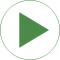# Information

This information is part of the Modelica Standard Library maintained by the Modelica Association.

This example demonstrates statistical properties of the Blocks.Noise.UniformNoise block using a uniform random number distribution. Block "noise" defines a band of 0 .. 6 and from the generated noise the mean and the variance is computed with blocks of package Blocks.Math. Simulation results are shown in the next diagram: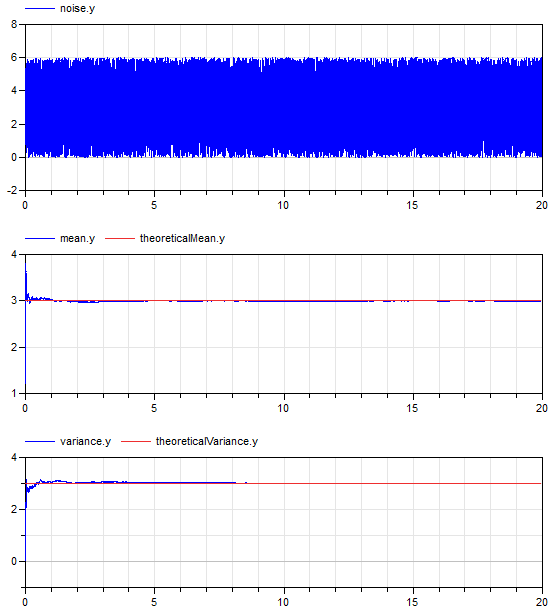The mean value of a uniform noise in the range 0 .. 6 is 3 and its variance is 3 as well. The simulation results above show good agreement (after a short initial phase). This demonstrates that the random number generator and the mapping to a uniform distribution have good statistical properties.

# Parameters (5)

y_min Value: 0 Type: Real Description: Minimum value of band Value: 6 Type: Real Description: Maximum value of band Value: (y_min + y_max) / 2 Type: Real Description: Theoretical mean value of uniform distribution Value: (y_max - y_min) ^ 2 / 12 Type: Real Description: Theoretical variance of uniform distribution Value: sqrt(var) Type: Real Description: Theoretical standard deviation of uniform distribution

# Outputs (2)

meanError_y Default Value: meanError.y Type: Real Default Value: sigmaError.y Type: Real

# Components (11)

globalSeed noise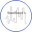Type: GlobalSeed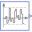Type: UniformNoise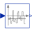Type: ContinuousMean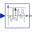Type: Variance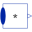Type: MultiProduct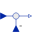Type: Feedback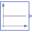Type: ConstantType: FeedbackType: Constant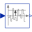Type: StandardDeviationType: Feedback